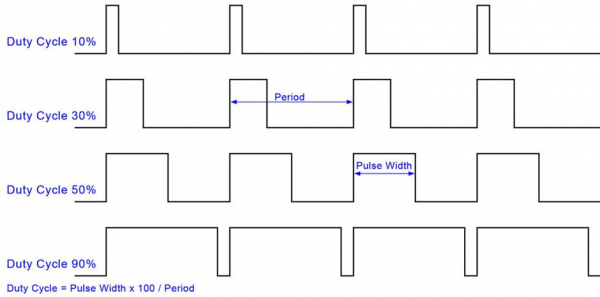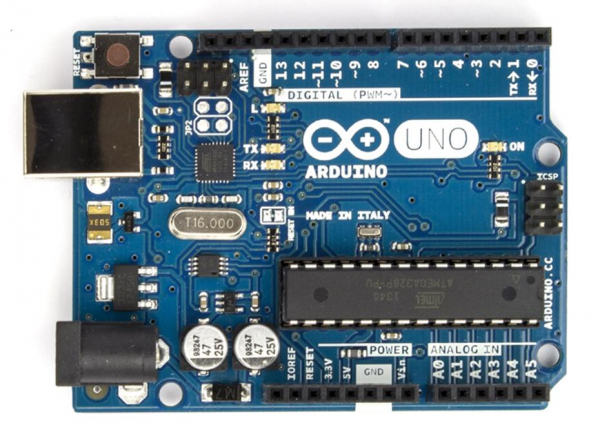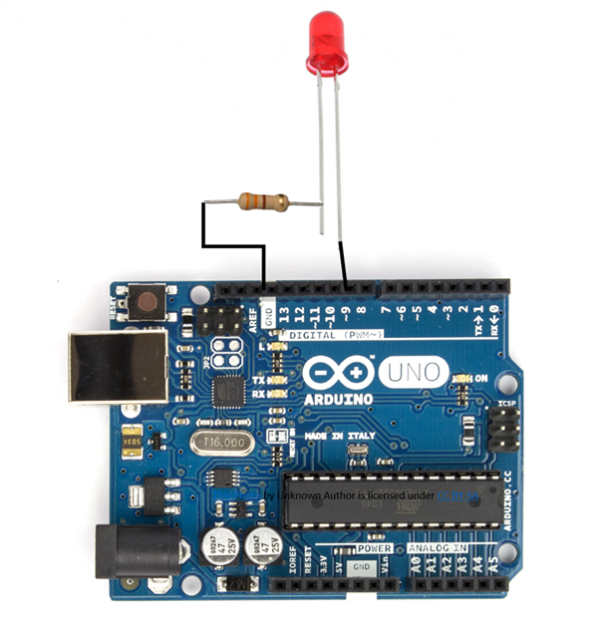# PWM in Arduino

## What is PWM?

PWM refers to Pulse Width Modulation. In very simple terms, we can output a square wave from certain pins of the Arduino board, and we can control the fraction of time for which the wave will be at the HIGH state (known as the duty cycle).## Why is PWM needed?

PWM finds several applications. An important application is running motors. The RPM of the motor can be controlled by the PWM output. PWM can also be used in general for generating voltages between HIGH and LOW. So, if your HIGH level is at 5V and LOW level is at 0V, and you need 3.5V output, if you generate a square wave which stays at the HIGH state for 70% of the cycle, or, in technical terms, has a duty cycle of 70%, and if its time period is very less (in milliseconds), then the node receiving this square wave will experience a voltage of 3.5V. With this application in mind, it shouldn’t be a surprise that the function used to perform PWM in Arduino is called analogWrite(). After all, we are essentially outputting analog voltage, instead of reading it.

## Which pins of Arduino support PWM?

Not all pins of Arduino support PWM. On the Uno board, the pins are specifically marked with a ~ symbol. For other boards, please refer to the datasheet of the board.As can be seen from the above picture, only pins 3,5,6,9,10 and 11 can support PWM. The frequency of the square wave is 490 Hz (about 2 ms time period) on all pins except 5 and 6, on which it is 980 Hz (about 1s time period).

## Example application

We will consider the LED brightening followed by dimming example. We will connect an LED to a PWM pin, and keep increasing the duty cycle on that pin, thereby increasing the voltage on that pin. Thus, the LED will keep getting brighter, till the max duty cycle (100) is reached.

Now, note that an 8-bit number is used to represent the duty cycle. Thus, the duty cycle can vary from 0 to 255. Thus, the value of 255 corresponds to duty cycle of 100%. 127 represents ~50% duty cycle, and so on.

The syntax for analogWrite is −

## Syntax

analogWrite(pin_number, duty_cycle)

The circuit diagram is shown below −Note: The longer leg (+ve) of the LED is connected to pin 9, the shorter leg (-ve) is connected to GND via a resistor (the resistor value can be of the order of 100 Ohms. We chose 220 Ohms).

The code is given below −

## Example

int led_pwm_pin = 9;
void setup() {
pinMode(led_pwm_pin, OUTPUT);
}
void loop() {
//Brightening the LED
for(int i=0; i < 255; i++){
analogWrite(led_pwm_pin, i);
delay(10);
}
//Dimming the LED
for(int i=255; i>0; i--){
analogWrite(led_pwm_pin, i);
delay(10);
}
}

As you can see from the code, we increase the duty cycle gradually from 0% to 100%, and then reduce it from 100% to 0%. You should see brightening followed by dimming on the LED.